# C2000 Solar MPPT tutorial Pt/2

In this second part of the C2000 Solar MPPT Tutorial, the hardware and circuit design will be looked at in greater depth.  The schematic for the system is posted again below for reference, which can be enlarged by simply clicking on it.## Buck Converter Design

The first step was to design the buck circuit, this is determined by the output parameters of the system and it’s load.  For the first prototype based around the panel purchased for testing, it was decided to aim for a 12V output, therefore a maximum current of 750mA (assuming 10% losses).

When calculating a buck circuit the frequency of operation, inductor size and output capacitor size are important, as they determine the current and voltage ripple size.  It is desirable to have as smaller current and voltage ripple as possible.  A large current ripple can cause additional losses in a system, as there maybe times when the peak current is greater than the load requirements.  A large voltage ripple is obviously not desirable, good quality regulated power supplies have very low voltage ripples.

A general rule is the higher the frequency the smaller the inductor and output capacitor size, and a smaller inductor and capacitor size generally lowers the system cost.  However higher PWM frequencies decrease the system efficiency due to switching losses in the mosfets, so a trade off has to be reached which meets the design constraints of the end system.

For this system a PWM frequency of 15kHz was chosen, based on this, the solar panel and other design parameters for the buck circuit calculations can be performed.  First we can determine the system duty cycle at MPP, note the duty cycle will change to track the MPP with differing irradiance.  A figure of 90% was used for the buck converter efficiency,a typically buck converter efficiency is 90% or greater.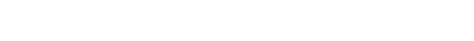Next an ideal current ripple can be determined, it is important to note that the below formulae used only determines a single phase current ripple.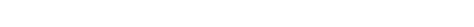ΔIL is the inductor ripple current, and in this case a 30% figure was used for the multiplier. Now that the current ripple is know the inductor size can be calculated with the following equation.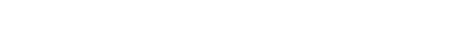Using two 1.34mH inductors on each phase will ensure the inductor ripple current is effectively halved, knowing this an inductor ripple current of 90mA can be used to calculate the minimum output capacitor size.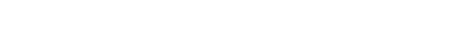Δvout is the desired ripple voltage.  The constant 8 is determined by the simplification of an equation, which can be found in various sources, one such source is an Application Note by On-Semiconductor AND9135/D.

There are two other factors for the inductor and capacitor that are important to consider: the inductor peak current and the capacitors Equivalent Series Resistance (ESR).  The inductor for this project will be hand wound using a toroidal core, which will be covered shortly.  The capacitors ESR can affect the reliability of the capacitor.  A capacitor will dissipate power as heat depending on it’s ESR, so a low ESR is desirable as excessive heating will shorten the life of a capacitor and be less efficient.  For this early prototype, cheap off the shelf capacitors were used as their reliability over time was not a concern at this stage.

The inductors were constructed using a T68-26A toroidal core, this core has a nominal inductance or Al value of 58nH.  The following equation was used to determine how many turns of wire the core would need.A 0.3mm outside diameter enamelled cable was chosen, this has a maximum current rating of 1.4A.  Then a very useful website found here was used, this allows you to calculate the total length of cable required based on the toroidal core dimensions and cable diameter. Using a vice the cores were both wound and then measured using a LCR meter and measured at 1.3mH, an image below shows the hand winding process used.## MOSFET Losses

For the prototype system Vishay IRFI640G MOSFETs were used, these are not the most efficient having a high RDSON value (180mΩ), but they were stocked at the time of writing.

The power losses from the High Side and Low Side MOSFETs are a combination of conduction and AC switching losses.  The conduction losses are a result of I²R losses inside the MOSFET when it is fully on, and the switching losses are the result of the MOSFET transitions from its on and off states.  Some example calculations will now be shown using data from the IRFI640G datasheet and various sources, on a synchronous MOSFET buck circuit efficiency.The first equation is for the High Side MOSFET and is based on the Vmp of the solar panel at maximum output current running through each interleaved phase.The next equation is for the Low Side MOSFET using the same current figure.The results from these two equations can be combined to work out the overall efficiency, the losses will also be multiplied by a factor of 2 as this is for a two phase interleaved circuit.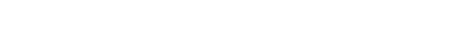Now if we increase the PWM frequency to 150kHz, the circuit losses will also be shown to increase.The switching losses in this next example will be shown to increase with increasing current, the next example uses a 15kHz switching frequency but with 2A, increasing the overall power to 24W.A MOSFET with a lower RDS(ON) will have lower conduction losses, but it will typically have a higher QG (Gate Charge) resulting in higher switching losses.  Therefore a careful balance between these characteristics should be found to maximise the circuits efficiency.  Taking into account the nominal parameters of the system, such as input voltage, output current, switching frequency and duty cycle, will allow the best efficiency to be achieved.  This will often involve using MOSFETs with different characteristics for the High and Low Side drivers.

## MOSFET Driver Circuit

The Half-Bridge (H-Bridge) driver is an IC designed specifically for driving MOSFETs. The IC takes the incoming PWM signal, and then drives two outputs for a High and a Low Side MOSFET.  This type of H-bridge is often used to to drive motors, but has other applications such as the following example.  The IC used is a IRS2003 half bridge driver made by International Rectifier, the image below shows an example circuit from the datasheet.The IRS2003 allows 2 PWM signals to be connected to HIN and LINNot this gives the user the opportunity to fine tune the dead-band switching of the MOSFETs.  The capacitor wired between VB and VS along with the diode form a charge pump, this allows the drive voltage to the MOSFETs to be almost doubled.  HIN and LINNot in this case are wired together and supplied with the same PWM signal, the IRS2003 has internal timing to ensure the High Side MOSFET, and the Low Side MOSFET are never on at the same time.  The capacitor between VB and VS needs to be sized to ensure it can drive enough current to the gate of the chosen MOSFET, over coming the gate capacitance.

There are four ADC ports used on this project, two sampling voltage and two sampling current.  The hardware employed to sample the voltage signals will be covered first, followed by the current sampling circuit.  But first a brief introduction to the TMS320F28027 ADC.

The ADC measures voltage from 0V to 3.3V, with a 12bit resolution.  It is important to not exceed the input voltage of the microcontrollers GPIO pins, the TMS320F28027 has a maximum input voltage of 3.63V.  Using this information the step resolution for the ADC can be calculated.The input on the ADC also has a small internal capacitance and resistance, this is used for Sample and Hold acquisition depending on the characteristics of the circuit being sampled. The internal ADC circuit taken from the TMS320x2802x datasheet is shown below.To ensure the readings being sampled are as accurate as possible, the source resistance or RS shown in the above image ideally needs to be as small as possible.  This will be achieved by placing an opamp configured as a unity gain buffer in all the ADC sample circuits.  The unity gain buffer will ensure a high input impedance, therefore reducing loading effects on the sampled circuitry to a minimum, as well as offering a very low output impedance to the C2000 internal ADC circuit.  Rail to rail opamps were used and supplied with 3.3V, this ensured the voltage passed to the ADC would not exceed this, thus ensuring the system has ADC protection built in.

The voltage sampling circuits consist of a simple potential divider, the maximum voltage the solar panel can produce is 21.6V when open circuit.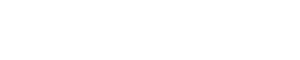The same resistor and opamp configuration is used for the input and output voltage measurement.  A more complex opamp circuit could have been used with offset, to fully exploit the range of the ADC, however this would provide more than enough accuracy for the prototype.

The current sampling circuit involved a slightly more complex approach.  The circuit would revolve around a Texas Instruments INA138 High Side Measurement current shunt monitor. The INA138 is basically a differential amplifier housed inside a small package, with a wide operating voltage.  The INA138 would be supplied with 12V, this then allows for a greater range to be measured around the 0V-3.3V range and was also the second supply voltage available for this circuit.  A typical configuration taken from the datasheet is shown in the image below.There are differences in input and output current so two formulas would be needed to ensure the range was correct.  The shunt resistors comprised of two 1Ω (1%) resistors in parallel, so the combined value becomes 0.5Ω.  The parallel resistors were measured and the actual value was approximately 0.47Ω.  A quick calculation to check these could withstand the power loads was made.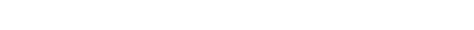This was not the best long term solution, but within tolerance for the 1% resistors in parallel.

The shunt resistor is connected directly across the internal differential amplifiers inputs. The calculations for the INA138 are fairly simple, the gain resistor soldered externally determines the overall gain of the device.  The datasheet for the INA138 states that the device has a gain of 1 with a 5kΩ resistor, gain of 2 with a 10kΩ, gain of 5 with a 25kΩ and so on and so forth. The input and output current gain resistors were calculated as follows.These values would ensure the output from both the current feedback circuits falls in-line with the ADC input.

## Schematic Design and Layout

Certain aspects of the design were simulated in OrCad 16.6 first, to back-up the theory with simulation.  Then the design was taking over to EagleCad to enable faster prototyping.  Component symbols and foot prints where designed for all the non standard parts, ensuring these were all compatible with the LPKF milling machine used.  Once the schematic design was complete and the Electrical Rule Check (ERC) and Design Rule Check (DRC) were satisfactory, a schematic design was made for a two layer board.  The layout kept the PWM and digital switching side and analogue circuitry away from each other to avoid unnecessary noise.  The below image shows the final PCB prototype layout.The next part of this tutorial will go more in-depth into the C2000 software and Perturb and Observe algorithm, also including a downloadable version of the C code.

## 22 thoughts on “C2000 Solar MPPT tutorial Pt/2”

Hello Amit,

The MOSFETs I’m planeing to be use are the IRL60B216, which can sink 300A at 18V Vds. Is this selection appropriate considering the need for a maximum of .6A thourgh the switch? could it sink more current and damage the half bridge driver?

Hi,

i am using 100 khz as a switching frequency for buck regulator.
As per 1N138 datasheet: Gain can be between 1 and 100 and output load resistance(or the gain) will affect the measurement bandwidth.

Moreover Electrical characteristic states that:
if RL = 5 Kohm , F = 800 khz
if RL = 125 Kohm, F =32 khz

Does it mean that for 100 khz switching frequency, maximum gain that can be selected is 8 and thus max output load resistance of 1N138 is limited to 40 kohm ???
That means there is a direct relation of gain of the 1N138 with the switching frequency of the buck regulator. Am i right or i am missing something???

Hi,

I think you are referring to connecting the INA138 to an ADC, see Typical Applications (continued) on the datasheet page 12 and also 8.2.2.2 Detailed Design Procedure on page 13.

Cheers,
Ant

I was referring to the gain resister that can be connected to the output pin of INA138. I want to know that is there any limitation on the max value of R3 and R4 resister used in your design.

i mean to say R1 instead of R3. Moreover what is the value of R7 and R8 used, it’s NOT quite clear from the design.

Hi,

Thank you so much for this detailed explanation.

I want to duplicate the same model but for 20 amp rating.
I have calculated values of the component taking Fs = 200 Khz, Vin = 17.2 volt, Vout = 12 volt as per the formula explained in this tutorial.

D = 63%, delta iL = 0.3*20 = 6 AMP, L = 3.02 uH, Cout = 187.5 uF
I will be using L = 5 uH, Cout = 500 uF with some lower capacitor in parallel for low value of ESR in the design

Since I = 20 amp and Fs = 200 khz , i have carefully selected MOSFET as “IRFZ44N”

Can you please confirm weather the component selection are correct or do i need to change the values and MOSFET. I will be using MSP430g2553 microcontroller.

Thank you !

Hi,

Firstly sorry for not replying sooner as been away for a few weeks. The IRFZ44N should be ok with 20A as within the thresholds for the device, you will need to ensure a good heatsink though as the MOSFET is likely to produce ~7W of heat.

Cheers,
Ant

Thank you for the reply Ant..!!
I have doubt in interleaved synchronous buck regulator.
Since interleaved regulator uses two synchronous buck regulator in parallel, where both high side MOSFET should be switched ON simultaneously and both low side MOSFET should be switched ON simultaneously. moreover care should be taken that both high side and low side MOSFET should not be ON at the same time.

If i understood correctly then only one PWM signal is enough to drive both the MOSFET Driver IRS2003 as driver has internal feature of NOT making high side and low side MOSFET ON at the same time. Moreover same PWM signal will insure that both the high side MOSFET are ON at the same time and both low side ON at the same time.

MY DOUBT:
why you have generated two pwm signal separately and that too with 180 degree phase shift as we can see clearly from the graph.
If we feed first PWM signal to first driver and second PWM signal to second driver then won’t if affect the buck regulator working as both the high side MOSFET will NOT be ON at the same time?

May be i am missing something.

I hope you understood by question..

Thank you Ant.

Hi Balkrishna,

I am not going to write a long reply, if you have a read of this link it might help bring some clarity to the synchronous/interleaved circuit. LINK

Cheers,
Ant

4.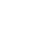hashimelahiprojects – I am electrical/ electronic engineer with a passion for the field. This is my hobby and like to share it with other people to contribute to the community. hashim elahi says:

Hi there,

The gate driver has the high side and low side pins shorted, which means both the high side and low side mosfet would switch on at the same time. This seems like a major fault or am I missing something?

Thank you.

Hi,

You need to correctly time the PWM signals to ensure the High and Low side are not on at the same time, but this circuit arrangement using a synchronous buck circuit is more efficient. Read part 1 of my tutorial for a basic explanation of why this is an improvement.

Cheers,
Ant

Hi,

Thanks for answering, but the problem is not about the buck converter, I may not have been clear about my question, sorry. It’s more about what happens before the buck converter, when the mosfet is OFF.

The thing is, when the mosfet is off, there is no current flowing from the solar panel to the buck converter. therefore, in the IV Curve of the PV cell, you end up in the I=0 point of the curve everytime your mosfet is in the off phase. Isn’t that a problem when you’re trying to track the MPP?

Therefore I’m wondering how do you manage to make your solar panel work at the MPP. Do you put something between your solar panel and your buck converter ? How do you choose the Vin for your buck converter ?

Hi,

The switching of the MOSFET is happening many thousands of times a second, so any effect is negligible. Additionally there are some small capacitors on the PV panel input. If this rate of switching was slowed down enough, say to 1Hz or something like that, then it would be like disconnecting the load for a longer period of time. In this case I would expect the PV voltage to rise and if the switching was off long enough, the voltage should in theory rise to the panels VOC characteristic.

Regards,
Ant

Hi,

How do you manage not to brutally go to 0V everytime the Mosfet is OFF? I guess you’d put a capacitor before the Buck, but how would you take it in consideration then?

Sorry, i meant 0 Amp, not 0 volts. (since the interruptor aka mosfet is off you’d get no current)

Hi,

The buck circuit has charged stored in the inductor, this then provides a source of power in the off phase of the MOSFET. I give a brief overview of the buck circuit operation in Part 1, which is worth reading. However, it would be worth looking up further literature on buck circuits for a more deeper understanding of the operation.

Cheers,
Ant

Hi,

the capacitances of output capacitor are 100uF, 3,3uF, 100nF. right?

could you explain why you use three capacitor though Cout_min = 75uF.

Thanks agian

Hi,

The values used were mainly due to stocked parts which were fairly cheap components, additionally I also had no datasheets available for ESR values etc. When making a design it is better in some cases to use smaller value capacitors in parallel as opposed to 1 large capacitor, as you can get a reduced ESR, also ceramic capacitors offer some advantages over Electrolytics which maybe desirable for the design. There are no calculations for the values I used except that they met or exceeded the minimum output capacitance I calculated for the buck converter design.

Best regards,

Ant

Thanks much for information!

I have a question.
In a schematic, T272 means that TLC272 made by TI??

Thanks again!